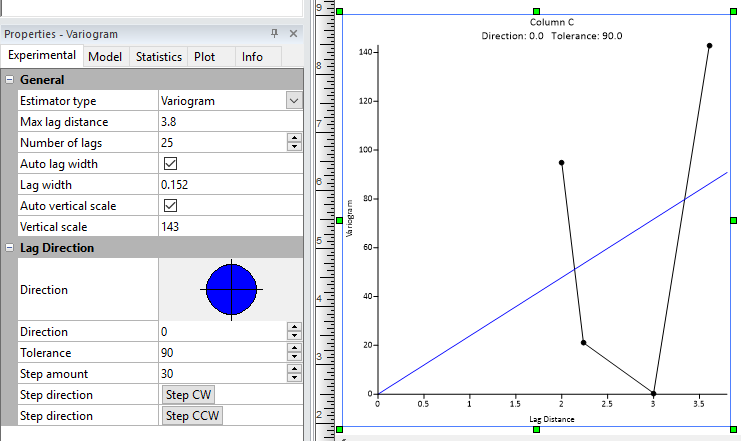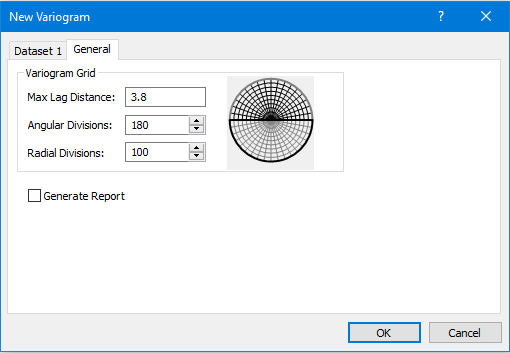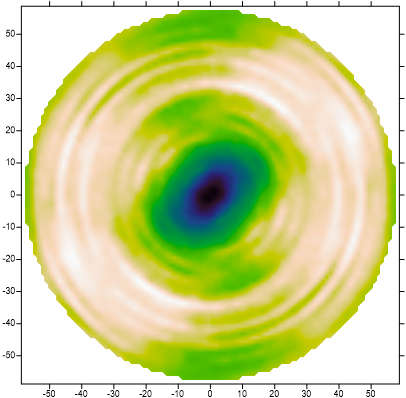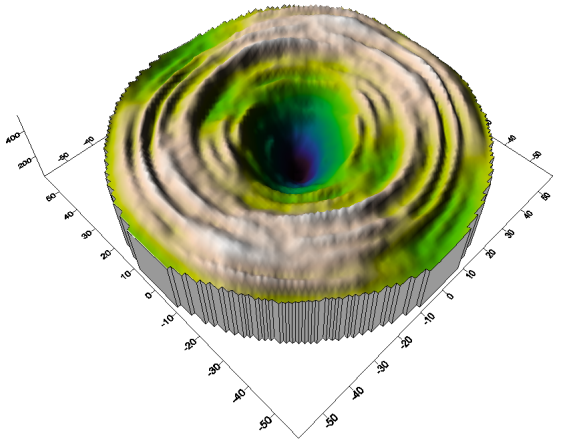Follow

# Creating a Variogram Map and Surface in Surfer

This article discusses variograms, how to create them, and how to plot maps of them in Surfer.

The Variogram Plot and Grid

A variogram displays half the average difference in Z values of two data points as a function of the separation distance between the points. The black curve displays the actual data, and the blue curve displays a function that models the data. The kriging gridding method uses the variogram model to calculate Z values throughout the map area.The variogram displays half the average difference between two points
as a function of their separation distance.

Surfer uses a polar grid to store a summary of variogram information for all the data pairs that fall into each grid cell (defined by the lag distance and angle). When the Tolerance (in the Lag Direction section) is set to less than 90°, the variogram curve shows the values for a particular direction through the variogram grid. The variogram grid is not directly accessible, but you can export it one line at a time. The angle of the Direction and magnitude of the lag can be used to calculate the XY coordinates, with the variogram value as the Z value. Grid the resulting XYZ file to display an image map or 3D Surface of the variogram data. The process can be automated with a script.The Surfer variogram grid stores information about the variance
between data point pairs based on the distance and angle between points.

Automating the Process with a Script

Exporting the variogram curves can be automated with a script. Refer to the attached VarioExport.BAS. The data set used in this script is Demogrid.dat which can be found in the Samples folder downloaded by default to C:\Program Files\Golden Software\Surfer <version>\Samples.

Manual Steps

1. Click the Grids | New Grid | Variogram | New Variogram command.
2. In the Open Data dialog, specify the data file, and click Open.
3. Click OK in the New Variogram dialog box to accept the default parameters.
4. Select Variogram in the Contents window.
5. On the Experimental tab of the Properties window, set the Direction in the Lag Direction section to 0.
6. Click the Grids | New Grid | Variogram | Export Variogram command.
7. In the Save As dialog, enter the file name variogram000.dat and click Save.
8. Click File | Open.
9. In the Open dialog, select your variogram DAT file and click Open. This opens the file in Surfer's worksheet.
10. Select row 1 and click Data | Edit | Insert to insert a blank row at row 1.
11. Type the following values into the columns of row 1:
column A = Lag
column B = Variogram
column C = # pairs
column D = X
column E = Y
12. To calculate the X and Y values, click the Data | Data | Transform command.
13. In the Transform dialog, change the First row to 2 so the header row is preserved, and then enter the following equation into the Transform equation box:

D = A*cos(d2r(0))

In this equation:

D = the destination column for the X values.
A = the lag or separation distance.
cos() = the cosine function.
d2r() = the degree to radian function.
0 = the lag angle for this variogram.
14. Repeat steps 12 and 13, entering this Transform equation into the box:

E = A*sin(d2r(0))
15. Click File | Save As to save the changes to a new file name (VarioCombined.dat).
16. Click back to the Plot1 window. Change the Direction in the Lag Direction section to 5 degrees.
17. Click the Grids | New Grid | Variogram | Export Variogram menu command and name the file variogram005.dat.
18. Click back to the VarioCombined.dat worksheet window.
19. Press the END key on your keyboard to go to the last row in the worksheet, and then click the down arrow key to go to the first empty row.
20. Click the Data | Edit | Merge command.
21. In the Import Data dialog, specify the variogram005.dat file and click Open.
22. Select the new rows.
23. Repeat steps 12-14 above to calculate the x and y values for these new entries, changing the angle in the Transform equations from 0 to 5:

D = A*cos(d2r(5))

E = A*sin(d2r(5))

24. Click File | Save the changes to VarioCombined.dat.
25. Repeat Steps 16 - 24 in 5 degree increments through 175 degrees.
26. To add the data from 180 through 360 degrees:
1. Press END on your keyboard, select the last row, scroll up, press SHIFT on your keyboard, and select the first row.
2. Press CTRL+C to copy the selection.
3. Scroll back to the end of the file, and select the first empty cell in column A.
4. Press CTRL+V to paste the selection.
5. Click Data | Data | Transform.
6. In the Transform dialog, enter this Transform equation then click OK:

D=D*-1

7. Repeat the previous two steps, using the Transform equation

E=E*-1

8. Click File | Save to save the changes.
27. Back in the Plot1 window, use the Home | Grid Data | Grid Data command to grid the data, and then create a color relief map, surface map, or contour map using the applicable command in Home | New Map.Color Relief map of the variogram data.3D surface map of the variogram data.